# JP3596339B2 - Inter-vehicle distance measurement device - Google Patents

## Info

Publication number
JP3596339B2
JP3596339B2 JP6839099A JP6839099A JP3596339B2 JP 3596339 B2 JP3596339 B2 JP 3596339B2 JP 6839099 A JP6839099 A JP 6839099A JP 6839099 A JP6839099 A JP 6839099A JP 3596339 B2 JP3596339 B2 JP 3596339B2
Authority
JP
Japan
Prior art keywords
distance
vehicle
edge
inter
image
Prior art date
Legal status (The legal status is an assumption and is not a legal conclusion. Google has not performed a legal analysis and makes no representation as to the accuracy of the status listed.)
Expired - Fee Related
Application number
JP6839099A
Other languages
Japanese (ja)
Other versions
JP2000266539A (en
Inventor

Original Assignee

Priority date (The priority date is an assumption and is not a legal conclusion. Google has not performed a legal analysis and makes no representation as to the accuracy of the date listed.)
Filing date
Publication date
Application filed by 日産自動車株式会社 filed Critical 日産自動車株式会社
Priority to JP6839099A priority Critical patent/JP3596339B2/en
Publication of JP2000266539A publication Critical patent/JP2000266539A/en
Application granted granted Critical
Publication of JP3596339B2 publication Critical patent/JP3596339B2/en
Anticipated expiration legal-status Critical
Application status is Expired - Fee Related legal-status Critical

## Images

•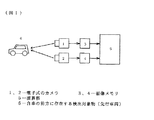•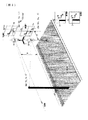•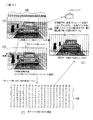•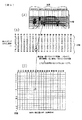•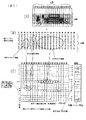•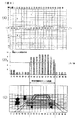•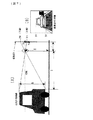•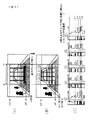•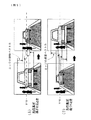•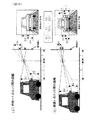•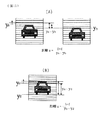•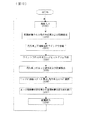•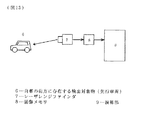•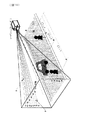•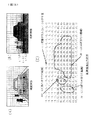•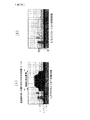•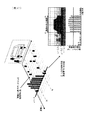•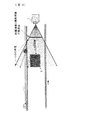•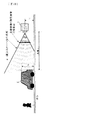•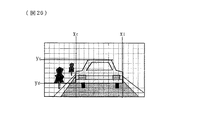•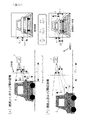•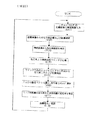•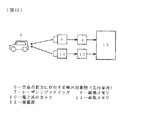•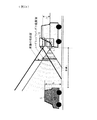••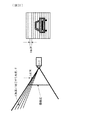•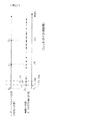•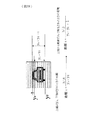•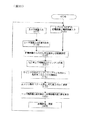## Description


TECHNICAL FIELD OF THE INVENTION
The present invention relates to a technique for improving the reliability of measurement of a following distance and a following distance change of a preceding vehicle that is following.

[Prior art]
2. Description of the Related Art As a conventional inter-vehicle distance measuring device, for example, there is one described in Japanese Patent Application Laid-Open No. 8-278126. In this apparatus, histograms of horizontal edges are extracted from vertically arranged stereo images, and the peaks of the histograms are searched in order from the lower side of the y-axis when the vertical direction is the y-axis. The inter-vehicle distance is obtained by using the difference in position between the stereo images on the y-axis as the parallax of the preceding vehicle.

[Problems to be solved by the invention]
However, in the above-described conventional apparatus, since only the histogram of the horizontal edge is used as the data used for measuring the inter-vehicle distance to the preceding vehicle, it is insufficient to determine whether the horizontal edge component is the edge of the preceding vehicle. There is a problem that a corresponding point search may be performed using an edge other than the vehicle. In particular, if there are multiple long and strong lateral edges on the road surface, such as a pedestrian bridge or stop line, those edges are emphasized more than the preceding vehicle, so the distance to the edge other than the preceding vehicle may be calculated. Will be higher. Further, since the distance is calculated only by the calculation from the stereo parallax, the resolution is coarse, and there is no matching with other methods, so that it is not possible to judge an erroneous measurement of the distance caused by the stereo matching. There was a problem.

The present invention has been made to solve the above-described problems of the related art, and an object of the present invention is to provide an inter-vehicle distance measuring device that improves the reliability of measuring the inter-vehicle distance with a preceding vehicle in the inter-vehicle distance measurement. And

[Means for Solving the Problems]
In order to achieve the above object, the present invention is configured as described in the claims. First, in the invention described in claim 1,Light measurement that is mounted on a vehicle and scans and irradiates light two-dimensionally in a direction parallel and perpendicular to the road surface, and measures the reflection intensity of the light in each irradiation direction and the distance to the reflection surface of the light. A distance device,
A luminance image in which the value of each angle of the measured reflection intensity is an array of digital values, and a distance image in which the value of each angle of the measured distance is an array of digital values in an order corresponding to the luminance image. A memory for storing
An electronic camera mounted on the vehicle at a position and orientation where the optical axis and the scanning center axis of the optical distance measuring device are parallel,
A distance / position for measuring an inter-vehicle distance to a preceding vehicle based on the distance image, and obtaining a position where the preceding vehicle exists on the image of the camera based on a position where the preceding vehicle is measured on the distance image. Arithmetic means;
Window setting means for setting a plurality of vertically long windows including the left, right, upper and lower ends of the preceding vehicle imaged on the image of the camera based on the position and the distance obtained by the distance / position calculating means,
Histogram calculation means for obtaining a histogram of a horizontal edge for each y coordinate in a vertically long window in all of the plurality of windows;
Edge detecting means for detecting a y-coordinate position of a horizontal edge which is a peak value of a histogram obtained by the histogram calculating means detected on the same y coordinate in the plurality of windows;
Vector measuring means for measuring a horizontal edge movement vector which is a peak value of a histogram detected on the same y-coordinate in a plurality of windows obtained by the edge detecting means;
Edge selection means for selecting only an edge indicating a movement vector of a direction and a size compatible with the change in the inter-vehicle distance obtained from the distance image as an edge of the preceding vehicle,
From the edges on the preceding vehicle determined by the edge selecting means, a plurality of pairs are selected as one set, and the distance between edges in each of the sets is determined. Preceding vehicle edge determining means for reconfirming the edge of the pair having the largest number of pairs in which the temporal change rate of the distance between edges, that is, the temporal change rate of the distance between edges, is the same as the edge on the preceding vehicle,
An inter-vehicle distance change rate calculating means for obtaining a change rate of the inter-vehicle distance based on a change rate of the inter-edge distance with respect to the edge on the preceding vehicle and the reconfirmed one;
An inter-vehicle distance calculating means for calculating an inter-vehicle distance by multiplying a calculated value of the inter-vehicle distance calculated by the distance / position calculating means a predetermined number of times before by a change rate of the inter-vehicle distance,
It is constituted so that it may be provided.
As described above, in claim 1, both an optical distance measuring device and an electronic camera mounted at a position and an attitude in which the scanning central axis and the optical axis of the optical distance measuring device are parallel to each other are used. The distance between vehicles is calculated by combining the luminance image of the optical distance measuring device and the image of the camera, and the window setting means is configured to set a vertically long window.

The invention according to claim 2The window setting means sets a horizontally long window, and the edge detecting means detects an x-coordinate position of a vertical edge which is a peak value of a histogram detected on the same x-coordinate. It is configured to.

Also,In the preceding vehicle edge determining means,A plurality of sets of two edges are selected as one set from the edges on the preceding vehicle determined above, the distance between edges in each of the sets is determined, and the distance between edges obtained at two points in time for the same set is determined. The configuration is such that the edge of the group having the largest number of pairs having the same value of the ratio, that is, the temporal change rate of the distance between edges, is reconfirmed with the edge on the preceding vehicle. For example, when ten edges are divided into five groups of a group, b group, c group, d group, and e group by two, the value of the change rate is α in four groups a to d, and only the e group is If it is β, it is determined that the edges of the groups a to d having the same value of the change rate are the edges on the preceding vehicle and the edges of the group e are other edges not on the preceding vehicle. I do.

Also,In the inter-vehicle distance change rate calculating means,The rate of change of the inter-vehicle distance is determined based on the rate of change of the inter-edge distance for an edge determined to be an edge on the preceding vehicle. Specifically, the reciprocal of the change rate of the inter-edge distance is the change rate of the inter-vehicle distance.

Also,In the inter-vehicle distance calculating means,The inter-vehicle distance is calculated by multiplying a calculated value of the inter-vehicle distance obtained by the distance / position calculating means a predetermined number of times before by the change rate of the inter-vehicle distance.

Claims3In the invention described in (1), the inter-vehicle distance is determined by comparing the inter-vehicle distance obtained by the distance / position calculation means with the inter-vehicle distance obtained from the change rate of the inter-vehicle distance.

Claims4The invention described inAnd the distance / position calculating means comprises:The preceding vehicle edge determining meansAtDo not use the measured value for the position determined as an edge other than the traveling vehicle, DistanceIt is configured to perform a calculation process of separation and position.

Claims5In the invention described in the above, the claim1Or claims2In this configuration, the distance / position calculation means performs the distance / position calculation processing without using the measured value of the position of the luminance image having a low value of the light ranging device.

【The invention's effect】
Claim 1And claim 2InBy combining an optical distance measuring device such as a laser range finder with a camera, it is possible to maintain or improve the distance accuracy even when the distance resolution of the optical distance measuring device is reduced in order to ensure reliability. Similarly, even if the scanning is delayed and the measurement time interval is lengthened in order to ensure the reliability of the device, even if a single camera is added, the response of the distance measurement will not be delayed, Distance measurement at the speed of image processing,The effect is obtained.

Claims 1 and 2, The temporal change rate of the distance between edges is determined, and an edge having the same change rate is determined as an edge of a preceding vehicle (an edge having a different change rate is removed as an edge other than the preceding vehicle). Therefore, it is possible to remove light noise and the like on the preceding vehicle, and it is possible to more reliably select only the edges constituting the preceding vehicle. Furthermore, by excluding edges other than the preceding vehicle as described above, the position of the excluded edge can be excluded when calculating the inter-vehicle distance. Even in an environment where there is much noise due to the position of light, it is possible to measure the distance between vehicles with high reliability without being affected by environmental changes.

Claims1 and claim 2, The change rate of the inter-vehicle distance is determined from the change rate of the inter-edge distance for the edge determined to be an edge on the preceding vehicle, so that there is some edge detection error such as light noise or shadow. In this case, and even in an environment where it is difficult to detect an edge on the preceding vehicle, the rate of change in the inter-vehicle distance can be accurately obtained.

Claims1 and claim 2In the method, the inter-vehicle distance is calculated by multiplying the calculated value of the inter-vehicle distance obtained by the distance / position calculation means a predetermined number of times before by the rate of change of the inter-vehicle distance, so that the inter-vehicle distance is higher than the inter-vehicle distance obtained from the parallax or the distance image. The distance between vehicles can be measured with high accuracy.In particularWhen applied to a configuration in which the optical distance measuring device and the camera are combined, even if the measurement interval of the optical distance measuring device is long, the inter-vehicle distance can be updated at the image capturing interval of the camera. Further, when the distance resolution of the optical distance measuring device is rough, the distance can be made more precise.

Claims3In the method, the accuracy of the inter-vehicle distance obtained by the two methods is confirmed by comparing the inter-vehicle distance obtained from the parallax or the distance image with the inter-vehicle distance obtained from the rate of change of the inter-vehicle distance by the distance / position calculation means. Since it is possible to perform the measurement, a more reliable inter-vehicle distance measurement can be performed.

Claims4smellAnd the distance / position calculating means comprises:Preceding vehicle edge judgment meansAtDo not use the measured value for the position determined as an edge other than the traveling vehicle, DistanceBy performing the calculation processing of the distance and the position, the certainty in the calculation of the distance and the position can be improved. For example, since the value on the distance image measured by the laser range finder at the position determined to be an edge other than the preceding vehicle is not reflected in the determination at the time of measuring the distance between vehicles, erroneous measurement values due to noise etc. can be removed, and Accurate ranging is possible.

Claims5In, claims1Or claims2In measuring the distance between vehicles from a distance image, the distance measured at a position where the reflection intensity on the luminance image of the optical distance measuring device is low is not reflected, so that an erroneous measurement value can be removed, and the reliability is further improved. More accurate ranging using only high measurement values is possible.

BEST MODE FOR CARRYING OUT THE INVENTION
(First Embodiment)
FIG. 1 is a block diagram showing the configuration of the first embodiment of the present invention.
In FIG. 1, reference numerals 1 and 2 denote electronic cameras, which are installed in front of the own vehicle so as to face forward. The optical axes of both cameras are parallel to each other, and the vertical axes of the imaging surfaces are the same. It is installed so as to be aligned with the top. In addition, you may install so that the horizontal axis of an imaging surface may be aligned on the same line. Further, it may be arranged such that the rear side of the vehicle is installed at the rear part of the vehicle to detect an obstacle behind the vehicle. Reference numerals 3 and 4 denote image memories for storing image signals input from the cameras 1 and 2, respectively. Reference numeral 5 denotes an arithmetic unit, which is configured by a microcomputer including a CPU, a RAM, a ROM, and the like, for example. Reference numeral 6 denotes a detection target such as an obstacle existing in front of the own vehicle, and FIG. 1 illustrates a preceding vehicle.

Hereinafter, first, various calculation means and methods used in the first embodiment will be described, and then the flow of the entire calculation will be described based on a flowchart.
FIG. 2 is a diagram for explaining the principle of obtaining the distance from the camera to the detection target based on the principle of triangulation using stereo images. In FIG. 2, an image captured by camera A (corresponding to the camera 1) is represented by an image A, an image captured by camera B (corresponding to the camera 2) is represented by an image B, and the position of the detection target is indicated by a point p (x , Y, z).

As can be seen from FIG. 2, the focal length f and the interocular distance (the distance between the two cameras) D are known, and two stereo images captured by two cameras A and B whose optical axes are parallel to each other are two. If the matching positions ya and yb between the images can be obtained, the distance Z from the camera to the object p can be obtained from the following equation (1).

Z = fD / (ya-yb) = fD / S (Equation 1)
Here, ya-yb = S is a parallax, and as shown in FIG. 2, when two cameras A and B having parallel optical axes and installed at a predetermined interval capture one object, The difference between the positions of the images captured by the camera, that is, the difference between the position ya in the image A and the position yb in the image B. In this example, the units of the interocular distance D and the distance Z are m, the focal length f, the parallax S, and the units of the positions ya and yb are pixels. For example, the cameras A and B use CCDs, and when the number of pixels is 640 × 480, the size of one pixel is about 10 μm.

The above equation (1) is a case where the cameras are installed such that the optical axes of both cameras are parallel to each other and the vertical axes of the imaging surfaces are aligned on the same line, but the horizontal axes of the imaging surfaces are on the same line. When they are installed so as to be aligned, the following (Equation 1 ′) is obtained.
Z = fD / (xa-xb) = fD / S (Equation 1 ')
However, xa−xb = S is parallax.
In the following description, an example will be described in which all the cameras are installed such that the vertical axes of the imaging surfaces are aligned on the same line.

In order to detect the parallax S, a point (xb, yb) on the other image (eg, image B) corresponding to a point (xa, ya) at which the point p is captured on one image (eg, image A). yb) needs to be detected. As a method for this, a range most similar to a certain range of images (windows) including a point (xa, ya) on the image A can be obtained by searching the image B for a range. The similarity is calculated by a difference method between images or a normalized correlation method. Then, the distance image (an image in which the parallax to the object imaged inside each window is obtained for each window) is a position where a window having a high similarity to the other exists in all defined windows by the difference method or the normalized correlation method. Can be created by asking for

FIG. 3 is a diagram showing the result of calculating the parallax for each of the corresponding positions of the two images. Specifically, in an image taken in front of the road, one image (for example, image B) is cut for each window, and all the images are cut. By obtaining the position of the image having the highest similarity with the other image (for example, image A) in the other window, the corresponding position in both images is detected, and the parallax of each window is determined from the corresponding position. It shows the result obtained. In FIG. 3, (A) is a lower image (corresponding to image A), (B) is an upper image (corresponding to image B), (C) is a table of parallax, and (D) is a window portion having parallax of “15”. Only the extracted image is shown. In addition, (1) to (20) in FIGS. 3B and 3C indicate positions of each window in the horizontal direction (hereinafter, referred to as a horizontal direction). However, in the figure, (1) to (20) are represented by circled numbers. Further, one window has a width (length in the x direction) xw and a height (length in the y direction) yw.
As described above, if the parallax of each window is known, the distance to the object imaged in the corresponding window can be obtained by using the above equation (1).

Hereinafter, an image obtained by calculating the parallax up to the object imaged inside each window as shown in FIG. 3C will be referred to as a “distance image”. The parallax calculated for each window is an edge (a portion where a point where an image changes from light to dark or dark to light is continuous and corresponds to a line segment indicating an edge of the image, etc.). ), The same parallax is obtained in adjacent windows if one target object is imaged over a plurality of windows. For example, in the case of a distance image in an image obtained by capturing the front of the road, the preceding vehicle and the road surface immediately below the preceding vehicle are at the same distance, and therefore, as shown by a thick window in FIG. The window on the same y-coordinate as is calculated with the same parallax as the preceding vehicle. For example, “15” in the second row from the bottom in FIG. 3C is continuous in the horizontal direction, which corresponds to the above-described portion. In FIG. 3C, the portion where the parallax “15” is gathered at the center corresponds to the preceding vehicle, and the portion where the parallax “19” is gathered in the rows (3) and (4) is “ The part where the parallax “5” is continuous in the (6) row corresponds to the “center tree”.

As described above, if an object having a height in the front exists in the distance image, the same parallax is detected in the window at the x-coordinate position where the object is imaged. On the other hand, at a position that does not have a height, such as a road surface, such as a white line portion next to the vehicle, only one window detects the same parallax on the same x coordinate. That is, when an object is present ahead, the object can be detected by counting the same number of parallaxes in windows in the same x-coordinate direction. According to this method, a plurality of objects and one object can be detected by the same method, and the object can be detected without being influenced by the detection target or the color of the background. In addition, the road surface display such as the white line and the stop line has an advantage that the windows showing the same parallax do not appear in the same direction, and thus the road surface display and an obstacle having a height are not erroneously detected. In addition, since only the distance image is used, a plurality of objects can be detected by the same processing regardless of the color or shape of the detection target and the background color.

FIG. 4 is a diagram showing a state in which voting is performed for a corresponding position in the table based on the parallax obtained in a certain window on the distance image and the horizontal position of the window. , (B) shows a parallax table, and (C) shows a voting table. Note that “voting” in this case means that +1 is added to a certain horizontal position and the position of the corresponding parallax value. For example, when one parallax “15” exists at the position (8), “+1” is added to the position of the parallax “15” at the position (8) in FIG. In the example of FIG. 4B, since there are five parallaxes “15” at the position (8), “5” is finally voted for the position of the parallax “15” at the position (8). Will be.

FIGS. 5A and 5B are diagrams showing the results of performing the above-mentioned voting in all the windows. FIG. 5A shows the upper image, FIG. 5B shows the parallax table, and FIG. 5C shows the voting table.

As can be seen from FIG. 4, in the windows set in FIG. 3, the windows at the same position in the horizontal direction are obtained by imaging the same direction. Also, as can be seen from FIG. 5, when an object is present ahead, the same parallax is obtained for the vertical window on the same x coordinate in the part where the object is detected, and when no object exists on the road surface, The same parallax is obtained in the horizontal window on the same y coordinate. When voting is performed on the table using the distance image in the method shown in FIG. 4, if the same parallax is arranged in the same direction (on the same x coordinate), the number of votes for the direction and the value of the parallax is determined. , The value at that position increases. Therefore, the presence or absence of an object ahead can be detected by searching for a position having a high value from the table of FIG. In the example shown in FIG. 5, the parallax “19” portion (corresponding to the left tree) in the (3) and (4) th windows, and the parallax “5” portion (the center tree) in the (5) th window ), (8) to (16), the voting is concentrated at the parallax "15" (corresponding to the preceding vehicle), and the value is high. For example, a high value of the parallax “15” portion indicates that an object having a parallax of about 15 pixels is captured within the angle of view captured by the camera. Assuming that the interocular distance D shown in FIG. 2 is 0.1 m and the focal distance f is 1500 pixels, the distance from the camera to the object is 10 m ahead (= 1500 × 0. 1/15). When the voting result at the position where the parallax is 15 pixels is viewed in the x-axis direction, the value near the (8) to (16) th windows defined in the x-axis direction is high, and the voting value is high in the left and right windows. It is lower.

FIG. 6 is a diagram showing a parallax 15 extracted from the voting result of FIG. 5 as a one-dimensional graph in order to clearly show the above contents. 6, (a) is a table corresponding to (c) of FIG. 5, (b) is a one-dimensional graph, and (c) is a corresponding image. Hereinafter, a method of obtaining an approximate horizontal (x-axis) range in which an object is imaged will be described with reference to FIG.

In FIG. 6B, the horizontal axis represents the position of the window defined in the x direction, and the vertical axis represents the number of windows for which parallax = 15 at the x coordinate position. In this figure, the (8)-(16) th values are high. This indicates that an object having a height between (8) to (16) is being imaged. The horizontal line in the graph is a threshold value provided for determining whether or not the object is imaged. The threshold value may be set, for example, by the following method. That is, the values in the table are proportional to the height of the object imaged on the image, and the height of the object on the image is inversely proportional to the distance to the object when the actual height of the object is constant. From this, it is possible to calculate the height on the image in accordance with the distance to the object, and set the threshold value of the histogram based on the number of windows included in the height. For example, in FIG. 6B, the threshold is set to 5.5. Therefore, since the position having a value equal to or larger than the threshold value is the (8)-(16) th window, the approximate horizontal range where the object in front is imaged is the (8)-(16) th window It is required to be between. The horizontal range in which the preceding vehicle is imaged as described above is xl to xr.

Next, FIGS. 7A and 7B are diagrams showing the relationship between the imaged positions of the upper and lower ends of the preceding vehicle captured in one image and the distance to the preceding vehicle. FIG. 7A is a side view, and FIG. 6 is an image example of a surface A.
When the distance Z to the preceding vehicle is known, and the height from the road surface to the camera is H and the height of the preceding vehicle is h, from FIG. 7, the upper and lower ends (yu, yd) of the preceding vehicle are almost equal. , Are captured at the positions of the following (Equation 2). Here, it is assumed that the lower end yd is almost the same height as the road surface.

yu = f × (h−H) / Z, yd = −f × H / Z (Equation 2)
FIG. 8 shows a state in which a vertically long window is cut on the preceding vehicle so as to include the upper and lower ends (yu, yd), and a histogram of a horizontal edge and a histogram of luminance are obtained for each y coordinate. 5A is a diagram illustrating an example of a vertically long window provided on an original image, FIG. 5B is a diagram illustrating an edge obtained by horizontally differentiating the location of a preceding vehicle, and FIG. The histogram of each horizontal edge of the window of (5) is shown. When a horizontally long window is set, a histogram of a vertical edge for each x coordinate is obtained.

The positions in the horizontal direction that define these vertically long windows may be between the ranges xl to xr in which the preceding vehicle detected from the distance image in FIG. 6 is imaged. Also, the vertical position defining the window is a size that includes the upper and lower ends yu and yd (the upper part is yu + α, the lower part is yd−α, α is a predetermined margin value). At this time, the height h of the preceding vehicle in the equation (2) is usually an unknown value, but considering a general vehicle height, an appropriate value in the range of about 1 to 2 m is sufficient. .

The preceding vehicle has a long lateral edge. Therefore, when a window is defined on the preceding vehicle, the peaks of the histograms of the horizontal edges appear at substantially the same positions in the windows defined at three places (2), (3), and (4) in the center of FIG. Here, from the distance image and the formula (2), since the window is provided only in the vicinity where the preceding vehicle can exist, the position (ye1) where the histogram peak exists at the same position between the windows in the defined window ~ Position of ye5) can be determined to be an edge on the preceding vehicle.

Next, the motion vector of the edge detected by the method of FIG. 8 is obtained, and only the edge that moves based on the motion of the preceding vehicle is selected from the edges.
9A and 9B are diagrams showing movement vectors of an edge, in which FIG. 9A shows an example in which the preceding vehicle moves away (the inter-vehicle distance increases), and FIG. 9B shows an example in which the preceding vehicle approaches (the inter-vehicle distance decreases). Is shown. As shown in FIG. 9, the edge on the preceding vehicle moves toward the center of the image (the position of y = 0 when the origin is the center of the image) in the y-axis direction when the inter-vehicle distance becomes large. When the distance becomes short, the image moves in a direction away from the center of the image. Therefore, if the edge detected by the method of FIG. 8 is an edge on the preceding vehicle, the edge at the position of y> 0 moves downward and the edge at the position of y <0 moves upward when the inter-vehicle distance increases. When the inter-vehicle distance is short, the edge at the position of y> 0 moves upward, and the edge at the position of y <0 moves downward, and when the inter-vehicle distance is constant, the position of the edge remains unchanged. Note that the movement vectors are indicated by vertical arrows in FIG.

The movement amount of the edge is larger as the distance from the origin is larger, and smaller as the edge is closer to the origin. From these facts, the change in the inter-vehicle distance is obtained by stereo image processing, the motion vector of the edge detected in FIG. 8 is obtained, and the vector whose motion moves as shown in FIG. The confirmation can improve the accuracy of the preceding vehicle detection. For example, an edge other than a vehicle, such as a road surface display immediately after a preceding vehicle or a pedestrian bridge ahead of the preceding vehicle, can be removed as an erroneously detected edge by the above processing.

Next, a method of determining the rate of change of the distance between edges and a method of reconfirming the edge on the preceding vehicle using the result will be described.
FIG. 10 is a diagram illustrating a relationship between the positions of the upper and lower ends of the preceding vehicle captured in one image and the distance to the preceding vehicle, where (A) is the position at time t−1 and (B) is Shows the position at time t.
As shown in FIG. 10, two pairs of lines parallel to the road surface, such as a bumper, are selected from different positions on the following vehicle, and the distances between the two parallel lines are defined as h1 and h2. The focal length is f, and the inter-vehicle distance is Z at time t-1.t-1The lengths of h1 and h2 on the image att, Y2tThen, y1t, Y2tAre respectively expressed by the following (Equation 3).

y1t-1= H1 · f / Zt-1, Y2t= H2 · f / Zt-1    … (Equation 3)
Further, the inter-vehicle distance at time t is ZtThen, similarly, the lengths of h1 and h2 on the image at that time are y1t= H1 · f / Zt, Y2t= H2 · f / ZtIt becomes. From these, the distance between vehicles is ZtTo Zt-1The temporal change rate (hereinafter simply referred to as a change rate) of the length between the edges on the image when the image is changed to is represented by the following equation (4) for both h1 and h2.

y1t/ Y1t-1= Y2t/ Y2t-1= Zt-1/ Zt    … (Equation 4)
As can be seen from the above equation (4), regardless of the distance between edges, the change rate of the distance between edges on the same object has the same value regardless of which edge is selected. Change rate (Zt/ Zt-1) (Zt-1/ Zt).
From this, two pairs are formed from the edges of the vehicle on the images detected by the methods of FIGS. 8 and 9, and the change rate of the distance between the edges (y1t/ Yt-1, Y2 // y2t-1, ..., ynt・ / Ynt-1) Can be determined, and from the rate of change of the distance between edges determined for each set, the edge of the most set having the same value can be reconfirmed as the edge on the preceding vehicle.

At the same time, the change rate of the distance between the edges obtained at the most places is the change rate of the size of the preceding vehicle on the image at that time. Furthermore, if there is a set that always has a different change rate only in a set including a certain edge, that edge can be excluded as an edge other than the preceding vehicle. As a result, not only the road surface display near the preceding vehicle and noise on the road surface, but also the noise of light that appears on the preceding vehicle but becomes a horizontal edge other than the preceding vehicle, such as reflected light from the outside, is also regarded as an edge other than the preceding vehicle. It can be removed.

When there is such an edge to be excluded, the value of the parallax at the position where the edge exists on the range image becomes an erroneous value. For this reason, in the distance measurement using the distance image, the certainty of the inter-vehicle distance measurement can be improved by not using the parallax of the existing position of the edge determined to be the edge to be excluded.
As described above, reliably selecting only the edge on the preceding vehicle by the determination as shown in FIGS. 9 and 10 is an effective means for increasing the certainty of the distance measurement. This is effective for improving the reliability of distance measurement in an environment where many edges other than the preceding vehicle are detected, such as ambient noise.

Next, a method for obtaining the rate of change of the following distance using the method described with reference to FIGS. 9 and 10 will be described.
The change rate of the edge-to-edge distance obtained in FIGS. 9 and 10 is the reciprocal of the change in the distance as shown in Expression (4). From this, the reciprocal of the change rate of the inter-edge distance obtained for the most edges can be used as the change rate of the inter-vehicle distance at that time. In this method, not only two edges on the preceding vehicle but also as many as possible are obtained based on the peak of the histogram. Therefore, even when some edges are not detected due to light reflection or the like, other edges are detected. If the edge can be detected, the change rate of the inter-vehicle distance can be obtained without being affected by the edge. Adding this determination is an effective means for calculating a stable and reliable change in distance to an environment in which it is difficult to detect an edge on the preceding vehicle.

Next, measurement of the inter-vehicle distance will be described. There are the following three methods for measuring the distance between vehicles.
(1) In stereo image processing using continuously input images, the following formula (1) is applied using the parallax of the preceding vehicle, so that the inter-vehicle distance to the preceding vehicle is continuously and temporally determined. Can be sought.
(2) The rate of change of the inter-vehicle distance during the following of the vehicle can be obtained by the method described with reference to FIGS. Therefore, if following the same vehicle, the inter-vehicle distance Z measured from parallax by stereo image processing several times agot-1Is multiplied by the reciprocal of the rate of change of the distance between the edges of the vehicle on the image several times before, it is also possible to calculate the distance between the vehicles at that time.
(3) A method of calculating the inter-vehicle distance from the height (yu-yd) of the preceding vehicle on the image.

Hereinafter, the method (3) will be described in detail. The height h of the preceding vehicle shown in FIG. 7 is usually an unknown value. However, if the distance Z to the preceding vehicle obtained by the stereo image processing and the height (yu-yd) of the preceding vehicle on the image are obtained, the height h of the following vehicle is calculated by the following equation (5). Can be requested.

h = Z × (yut-Ydt) / F (Equation 5)
Where f: focal length
yut: Value of yu at time t
ydt: Value of yd at time t
Here, since only the edge of the preceding vehicle can be detected with high certainty by the methods of FIGS. 8, 9 and 10, of the combinations of edges on the preceding vehicle detected from the image, the uppermost edge and the lowermost edge are combined. , It is possible to obtain the height (yu-yd) of the preceding vehicle on the image. Since the height h of the preceding vehicle during the following is constant, if the vehicle is following the same vehicle, once the height h of the preceding vehicle is obtained, the height h of the image newly detected based on the h is calculated. Height of preceding vehicle (yut + 1-Ydt + 1) Is substituted into Expression (5) to calculate the inter-vehicle distance Z from the height of the preceding vehicle on the image (the distance between the edges on the preceding vehicle) as shown in Expression (6) below. You can do it.

Z = h · f / (yut + 1-Ydt + 1)… (Equation 6)
Where h is a value calculated by equation (5).
Substituting h in equation (5) into equation (6) gives equation (6 ') below.

Zt + 1= Zt× (yut-Ydt) / (Yut + 1-Ydt + 1)
Or ... (Equation 6 ')
Zt= Zt-1× (yut-1-Ydt-1) / (Yut-Ydt)
Where Zt + 1: Distance between vehicles at time t + 1
Zt: Distance between vehicles at time t
Zt-1: Distance between vehicles at time t-1
yut-1: Value of yu at time t-1
ydt-1: Value of yd at time t-1
As can be seen from Expression (6 '), the inter-vehicle distance obtained by the above method is the inter-vehicle distance Z at time t obtained by stereo.tTo the reciprocal of the rate of change of the distance between edges on the image (yut-Ydt) / (Yut + 1-Ydt + 1) Is equivalent to multiplying In other words, the methods (2) and (3) are different in the calculation formulas to be actually applied, but are the same in principle.

The distance measurement methods (2) and (3) can increase the accuracy of distance confirmation and distance measurement. That is, the confirmation of the distance can be performed by comparing the distance calculated in the stereo image processing of (1) with the distance obtained from the change rate of the distance between edges in (2) or (3). The inter-vehicle distance change rate obtained from the inter-edge distance change rate is more accurate than the inter-vehicle distance change rate calculated from the distances continuously obtained by the stereo image processing.

Here, the reason for the improvement in accuracy will be described with reference to FIG.
Consider the difference in the accuracy of the inter-vehicle distance obtained by the method (1) and the methods (2) and (3). In the case of an in-vehicle stereo camera, since h> D (h: height of a preceding vehicle, D: distance between cameras), parallax (ya-yb) (unit: pixel) when the same inter-vehicle distance is measured is calculated. On the other hand, the height (yu-yd) (unit: pixel) of the preceding vehicle has a larger value. Therefore, the inter-vehicle distance measurement by the formula (6) has a smaller influence of an error due to a shift of one pixel, and thus enables a distance measurement with a finer resolution than the inter-vehicle distance measured from stereo parallax.

For example, let us consider a case where the inter-vehicle distance is 10 m when the inter-eye distance D is 0.2 m, f is 1000 pixels, and the height of the preceding vehicle is h = 1 m. At this time, the stereo parallax of the preceding vehicle is 20 pixels (= 0.2 × 1000/10), and the height of the preceding vehicle on the image is 100 pixels (= 1 × 1000/10). In these two cases, if the parallax and the detection of the height of the preceding vehicle are shifted by one pixel, in the case of stereo parallax, 20 pixels become 19 pixels, so that it is measured as 10.52 m (= 0.2 × 1000/19). On the other hand, in the height measurement, 100 pixels are 99 pixels, so that the height is measured as 10.1 m (= 1 × 1000/99). That is, the detection error of one pixel is 0.5 m in the stereo image processing, but is 0.1 m in the height measurement.

The displacement of one pixel for detecting the parallax and the height on the image corresponds to the inter-vehicle distance resolution to be measured. In other words, in the case of inter-vehicle distance measurement using an in-vehicle stereo camera, it is practically difficult to set a camera having an inter-eye distance larger than the height of the preceding vehicle. Measurement can be performed with finer resolution than measurement using stereo parallax. From this, obtaining the inter-vehicle distance to the preceding vehicle following the vehicle by two methods and comparing the results will not only confirm the inter-vehicle distance but also increase the accuracy of the inter-vehicle distance obtained from stereo parallax. Will be. For the same reason, the rate of change of the inter-vehicle distance is more accurate when calculated from the change in the inter-edge distance on the image than when calculated from the inter-vehicle distance obtained by stereo image processing. From this, the methods (2) and (3) enable confirmation of the inter-vehicle distance obtained by the stereo image processing and higher accuracy.

The above description has been made on the case where the two cameras are installed such that the optical axes are parallel to each other and the vertical axes of the imaging surfaces are aligned on the same line, but the horizontal axes of the imaging surfaces are on the same line. It may be installed so as to be aligned. In the description of FIG. 8, a case has been described in which a plurality of windows for edge detection set on the preceding vehicle are vertically long, but a horizontally long window is used, and a target of an edge on the preceding vehicle to be detected is a vertical edge. The same principle can be applied to this. Similar effects can also be expected regardless of how the camera is placed and the direction of the detection target edge.

Next, an embodiment will be described in which an edge on a preceding vehicle being followed is detected using the method described above, and a change rate of the inter-vehicle distance is determined to confirm the inter-vehicle distance and improve the accuracy. Here, as shown in FIG. 2, a stereo camera is used in which the cameras are arranged vertically parallel to the road surface and the two cameras are arranged so that the y axes of the two cameras are on the same line.

FIG. 12 is a flowchart showing the flow of the arithmetic processing in this embodiment.
First, in step S101, stereo images of cameras A and B in FIG.
Next, in step S102, a distance image is created by obtaining parallax, and the position and distance of the preceding vehicle are detected from the created distance image (see FIGS. 4 to 6).

Next, in step S103, first, a range (x1 to xr) in the lateral (x-axis) direction in which the preceding vehicle exists is obtained from the distance image (see FIGS. 6 and 8). Further, based on the distance Z to the preceding vehicle obtained from the distance image, the approximate upper and lower ends yu and yd of the preceding vehicle are obtained by the above equation (Equation 2), and a vertically long window having a size including yu and yd is obtained. (See FIGS. 6 to 8).
It is sufficient that the size of the window in the horizontal direction is about 10 pixels or more so that a histogram for each y coordinate can be obtained. Alternatively, since the size of the image of the preceding vehicle on the image is variable according to the distance, the width of the window having a width of 10 pixels or more can be variable at an appropriate size so that it can be defined at five or more places according to the distance. Good.
An average value of about 1 to 2 m is sufficient for the height h of the preceding vehicle used when obtaining the definition positions yu + α and yd-α of the upper and lower ends of the window based on the expression (2). In addition, the value of the margin value α may be a margin of about one fifth of the height of the preceding vehicle according to the distance of the preceding vehicle at that time. For example, when the height of the preceding vehicle on the image calculated from the distance from the formula (2) is about 50 pixels, α may be about 10 pixels.

Next, in step S104, a histogram of the horizontal edge in the vertically long window defined in step S103 is obtained (see FIG. 8). At this time, as shown in FIG. 8B, the histogram of the horizontal edge may be obtained by horizontally differentiating the image in the window using a Sobel filter or the like, and obtaining a histogram for each y coordinate of the horizontal differential image.

Next, in step S105, a horizontal edge on the preceding vehicle is detected from these histograms. That is, from the histograms of the horizontal edges obtained in all the windows defined in FIG. 8, edges detected at the same position in more than half of the windows are found. Since the preceding vehicle has an edge parallel to the road surface, an edge detected at the same position in the window cut on the preceding vehicle can be regarded as an edge of the preceding vehicle.

If one window is defined on the preceding vehicle and the white lines are captured at both ends of the window, the edge strength of the white line is often stronger than the edge intensity of the preceding vehicle. Instead, the position of the white line may be detected. Therefore, in order to prevent such erroneous detection, a plurality of windows are provided, and edges detected in the plurality of windows are determined to be edges on the preceding vehicle.

Next, in step S106, the movement vector of the edge on the preceding vehicle obtained in step S105 is detected (see FIG. 9). The movement vector of the edge may be calculated by applying a general optical flow detection method at the position of the edge detected by the above-described method, for example, by obtaining the moving direction of a small window cut on the detection target. A motion vector for each edge detected from the optical flows of all edges detected by such processing is obtained.

In step S106, it is reconfirmed whether or not the edge is on the preceding vehicle by using the movement vector of the edge. This confirmation can be made based on the following criteria based on the inter-vehicle distance change and the position where the edge exists (see FIG. 9). First, when the inter-vehicle distance changes, the edge near the center of the image has a smaller motion, and the motion increases toward the edge of the image. Therefore, for example, if there is a vector that is closer to the position of y = 0 (the origin: the center of the image) but has a larger motion than the average motion of the edge vector at a position farther from the origin than the edge, it is the preceding motion. It is highly likely that it is an edge other than a car. Further, as shown in FIG. 9, the edges on the vehicle move upside down in the range of y <0 and the range of y> 0. It can be judged as an edge. Also, an edge that shows a large motion when the inter-vehicle distance change is 0 can be determined as an edge other than the preceding vehicle. Based on such a criterion, an edge having a high possibility other than the preceding vehicle is removed, and a remaining edge (an edge meeting the above criterion) is determined as an edge on the preceding vehicle. As a result, an erroneous edge such as a pedestrian bridge or light reflection can be removed, and the edge on the preceding vehicle can be reliably selected.

Next, in step S107, the change rate of the inter-edge distance is calculated, and the change rate of the inter-vehicle distance (equal to the reciprocal of the change rate of the inter-edge distance: see equation 4) is calculated based on the calculated change rate.
First, two pairs are formed from the edges on the preceding vehicle detected by the methods of FIGS. 8 and 9, and the distance between edges in all the pairs is determined (see FIG. 10). As described above, in any set of edges on the same object, the temporal change rate of the distance between edges (y1t/ Y1t-1, Y2t/ Y2t-1, ..., ynt/ Yn-1) should be the same value.

Here, a method of obtaining the change rate of the distance between edges will be specifically described. The edge selected based on the criterion in FIG. 9 is an edge for which a temporal movement vector is obtained by an optical flow. Therefore, the start point of the vector is the previous edge position, and the end point is the current edge position. That is, the previous distance between edges ynt-1(N: 1, 2, ...) is the distance between the start points of the movement vectors of the two edges for which the distance between edges is to be obtained, and the current distance between edges ynt(N: 1, 2, ...) is obtained as the distance between the end points of the movement vectors of the two edges. In this manner, the rate of change yn of the distance between edges in all setst/ Ynt-1If (n: 1, 2,...) Is obtained, it is possible to determine the edge of each group in which the same value is obtained most from the edges as the edge on the preceding vehicle.

The reciprocal of the change rate of the distance between edges is the change rate of the inter-vehicle distance at that time. In this method, not only the distance between the two selected edges but also many changes in the distance between the edges showing the same change rate are observed. Therefore, even if there is one or two edge detection errors, the change rate of the inter-vehicle distance is determined. Can be obtained correctly. Therefore, it is possible to improve the certainty of the measurement of the inter-vehicle distance change rate.
Next, in step S108, the inter-vehicle distance measured by the stereo image processing is reconfirmed based on the change rate of the inter-edge distance.
As described in Expression (4), the reciprocal of the change rate of the distance between edges is the change rate of the inter-vehicle distance. That is, when the change rate of the current inter-edge distance with respect to the immediately preceding edge distance is obtained, the inter-vehicle distance Z obtained from the parallax by the stereo image processing immediately before is obtained.t-1Is multiplied by the reciprocal of the change rate of the distance between edges to obtain the current inter-vehicle distance Z.tCan be calculated. Since the inter-vehicle distance calculation based on the parallax of the stereo image processing is also performed every time, the inter-vehicle distance obtained by the stereo image processing can be confirmed by using the change rate. Further, as described in FIG. 11, the change rate of the inter-vehicle distance obtained from the change rate of the inter-edge distance is higher in accuracy than the change rate of the inter-vehicle distance obtained from the inter-vehicle distance continuously obtained by the stereo image processing. Therefore, it can contribute to high accuracy of the measured inter-vehicle distance itself.

In addition, in the description of the equations (5) and (6), it has been described that the inter-vehicle distance can be calculated from the inter-edge distance by calculating the height h of the preceding vehicle following the vehicle. Practically, if it is determined that the edge used for obtaining the above h is an edge other than the preceding vehicle by the determinations in FIGS. 9 and 10, the distance between the other two edges is used. This is dealt with by recalculating h to a new value. As a result, erroneous measurement of the inter-vehicle distance calculation using the inter-edge distance can be prevented, and it is possible to cope with a change in the vehicle height h due to, for example, replacement of a preceding vehicle. That is, when the preceding vehicle is replaced, the vehicle height h changes discontinuously at that time, and thereafter becomes substantially constant. In such a case, it is determined that the preceding vehicle has been replaced, and What is necessary is just to perform a process.

As described above, in the first embodiment, the position of the preceding vehicle in the horizontal direction on the image is calculated from the distance image obtained from the parallax based on the image obtained by the stereo camera having the optical axes parallel to each other and installed on the vehicle. And the actual inter-vehicle distance to the preceding vehicle, and at the position on the image obtained from the distance image, the vertical (or horizontal) size of the size including the upper and lower ends of the preceding vehicle is calculated based on the inter-vehicle distance at that time. Multiple windows are cut, a histogram of horizontal edges at each y-coordinate of each window (or a histogram of vertical edges at each x-coordinate) is taken, and an edge detected at the same position in many windows among the defined windows. Is determined to be an edge on the preceding vehicle, and the motion vector of the edge is determined. By configuring only the edge indicating the movement vector of the magnitude as the edge of the preceding vehicle, without erroneously detecting strong edges other than the preceding vehicle, such as pedestrian bridges, white lines, road surface display, etc. The edge above the preceding vehicle can be reliably selected.

Further, since the temporal change rate of the distance between edges on the same object is the same at any distance between edges, the detected edges are grouped two by two, and the temporal change of the distance between edges in all the groups. By determining the rate of change and removing the set of edges having different rates of change as edges other than the preceding vehicle, it is possible to remove light noise and the like on the preceding vehicle, and to more reliably identify the preceding vehicle. Only the constituent edges can be selected.

Furthermore, by excluding edges other than the preceding vehicle by this method, the parallax of the position of the excluded edge can be excluded when calculating the inter-vehicle distance. The inter-vehicle distance can be measured with high certainty even in a noisy environment due to the location of light and light.

In addition, based on the change rate of the inter-edge distance described above, the change rate of the inter-vehicle distance at that time is obtained based on the change rate calculated with the same value in the largest number of sets. In the process using only the image of the vehicle, the change rate of the inter-vehicle distance can be correctly corrected not only when there is some edge detection error such as light noise or shadow, but also in an environment where it is difficult to detect the edge on the preceding vehicle. It is possible to ask.

In addition, by calculating the new inter-vehicle distance at the time of processing by multiplying the inter-vehicle distance obtained in the past by the stereo image processing by the rate of change of the inter-vehicle distance, the inter-vehicle distance obtained by the stereo image processing is calculated. It is possible to collate and confirm with the inter-vehicle distance. Furthermore, the rate of change of the inter-vehicle distance obtained from the rate of change of the inter-edge distance is more accurate than the rate of change of the inter-vehicle distance obtained from the inter-vehicle distance continuously obtained by stereo image processing. It can also contribute to higher accuracy.

(Second embodiment)
In the first embodiment, the description has been given of the case where the distance between vehicles is measured by stereo image processing using two cameras. However, in the second embodiment, a so-called laser range finder is used instead of the two cameras. A device for measuring the distance between vehicles by using the device will be described.

FIG. 13 is a block diagram showing a configuration of the second exemplary embodiment of the present invention.
In FIG. 13, reference numeral 7 denotes a laser range finder, which is installed at the front of the own vehicle so as to face forward. In addition, it is also possible to arrange so as to face the rear of the vehicle with the rear facing, and to detect an obstacle behind the vehicle. The laser range finder 7 is a device that two-dimensionally measures the distance to a forward object and the reflection intensity (luminance) of the irradiation target by scanning the irradiation of the laser radar vertically and horizontally. An image memory 8 stores an image signal input from the laser range finder 7, and stores a luminance image and a distance image described later. Reference numeral 9 denotes an arithmetic unit, which is configured by a microcomputer including, for example, a CPU, a RAM, a ROM, and the like. In addition, the same reference numerals as those in FIG.

Hereinafter, first, various calculation means and methods used in the second embodiment will be described, and then the flow of the entire calculation will be described based on a flowchart.
FIG. 14 is a diagram showing the positional relationship between the laser range finder 7 that scans the front of the road in the left, right, up, and down directions with the vehicle traveling as the center, and the measurement object (preceding vehicle 6). The laser range finder 7 is mounted so that the central axis of scanning is parallel to the road surface and faces the traveling direction of the host vehicle traveling straight.

FIG. 15 is a diagram showing an example of a distance image and a luminance image measured by the laser range finder 7 in FIG. 14, where (A) shows a luminance image as an image, and (B) shows a distance image. (C) is a diagram showing the content (numerical value: digital value, for example) of the distance image. The distance image means a two-dimensional array of measured distances for each angle measured by the laser range finder, and the luminance image means a two-dimensional array of the intensity of the reflected light. Although a numerical value table (actually a digital value) as shown in (C) is shown, the contents of the luminance image (numeric value table) are not shown. In FIG. 15C, “−1” indicates a location (for example, the sky) where the distance is out of the range of the measurable distance and cannot be measured. Numerical values such as “18”, “28”, and “40” indicate the distance (m) to the object.

The following description is based on the assumption that the horizontal axis of the image is the x axis, the vertical axis is the y axis, and the origin is the center of the image.
As shown in FIG. 14, the coordinates of the points on the luminance image and the distance image obtained here and the positional relationship between the points on the real space from which the points on the coordinates are measured, It is determined by the size of the irradiation angle (angular resolution) that moves at each sampling interval for measuring the value. For example, when the vertical and horizontal angular resolutions are θ and φ, respectively, the position in the real space corresponding to the position of the coordinates (x = 2, y = 3) on the image is the center axis of the laser range finder (image A point on the object plane at the closest position in the direction of 2θ horizontally and 3φ vertically from an axis perpendicular to the plane). Also, the measured values at the same coordinate position of the distance image and the luminance image are the measured values at the same position in the real space.

FIG. 16A is a diagram in which only the portion indicating the same distance as the preceding vehicle is indicated by a thick line in the distance image of FIG. When there is a preceding vehicle ahead of the road, as shown in the figure, the portion indicating the same distance as the preceding vehicle is a portion of the road surface on and immediately below the preceding vehicle. That is, at the position where the object exists, the values indicating the same distance are adjacent to each other. FIG. 16B shows a measurement result when there is no preceding vehicle ahead. As shown in the figure, when there is no object such as a preceding vehicle on the road, the road surface is imaged in the lower half area of the image (the upper half is empty), and the distance measured in this lower half area is displayed on the screen. From short distance to long distance from bottom to top. That is, when an object exists, pixels indicating the same distance are arranged on the same x coordinate, but when there is no object, there is no value of the same distance on the x coordinate. From this, it is possible to detect and measure an object by the method shown in FIG.

FIG. 17 is a graph showing values obtained by taking a distance in the z-axis direction and voting with the x-axis as the x-axis direction of the image in the y-axis direction. Based on the value of the distance image as shown in FIG. 15C, a vote is made to the corresponding position in FIG. The voting means, for example, an operation of adding y = 1 to x = 2 and z = 3 in a graph when a pixel on x = 2 and a distance is 3 m. The details are the same as those of the first embodiment described with reference to FIGS.

Such an operation is performed for the values of the entire distance image. When an object exists at the position of z in front, the voting is repeated a plurality of times at the position of the distance z of the x coordinate where the object is imaged, so that the value of the position in the table becomes high. On the other hand, on the x-coordinate where no object exists, the same distance is not measured on the same x-coordinate, as shown in FIG. From this, based on the voting result, the presence or absence of an object can be determined from the presence of a position with a large value in the table. Further, the position where the object exists is determined by the position in the x-axis direction of the range xl to xr (xl is the left end of the existing range and xr is the right end) in which the voting value is a predetermined value or more in the x-axis direction. The distance can be determined by the value of the distance z in the table.

Next, a method for obtaining the imaging range of the preceding vehicle from the luminance image will be described. The range of the imaging range in the x-axis direction is obtained as xl to xr by the above-described method. The range of the image in the y-axis direction is obtained based on the following principle. 18 to 20 are diagrams showing the relationship between the imaging range and the distance to the preceding vehicle in the distance image and the luminance image captured by the laser range finder, FIG. 18 is a plan view, FIG. 19 is a side view, and FIG. It is an image which shows the existence range of a preceding vehicle. Hereinafter, a method of obtaining the range in the y-axis direction will be described with reference to FIGS.

The position of the upper end of the preceding vehicle on the image is y = yu, and the lower end is y = yd. Assuming that the vertical angular resolution is φ, the distance to the preceding vehicle is z, the height from the road surface to the center axis of the laser range finder is H, and the height of the preceding vehicle is h, FIG. The upper and lower ends (yu, yd) of the preceding vehicle on the measured luminance image are
tan (yu × φ) = (h−H) / z, tan (yd × φ) = − H / z
It becomes the relationship. However, here, the lower end is considered to be almost the same height as the road surface. Since θ and φ are minute angles, tan θ ≒ θ and tan φ ≒ φ. From this, (yu, yd) is obtained by the following equation (7).

yu = (h−H) / (φ × z), yd = −H / (φ × z) (7)
According to the above method, the range on the image where the preceding vehicle is imaged can be obtained as shown in FIG. 20, with the upper left end being (xl, yu) and the lower right end being (xr, yd).

Next, a vertically long window is cut in the imaging range of the preceding vehicle on the luminance image obtained by the above method, and a horizontal edge on the preceding vehicle is detected. The positions in the horizontal direction at which these vertically long windows are set may be the ranges xl to xr in which the preceding vehicle detected from the distance image is imaged. The position in the vertical direction may be set to a size including yu and yd (yu + α above and yd−α below) based on Equation (7). The content of the method of detecting a horizontal edge using this is the same as that of FIG. 8 in the first embodiment and its description, and therefore will be omitted. When a vertical edge is detected, the same processing may be performed by cutting a horizontally long window and replacing the y coordinate with the x coordinate. The same applies to the calculation of the movement vector and the distance between edges described later.

Next, the motion vector of the edge detected by the above-described method is obtained, and only the edge that moves based on the motion of the preceding vehicle is selected from the edges, which is described in the first embodiment. 9 and its description are omitted here.

Next, a description will be given of a method of calculating the change rate of the inter-edge distance and the change rate of the inter-vehicle distance, and a method of reconfirming the edge on the preceding vehicle using the results. This method is similar to that of the first embodiment shown in FIG. 10 and the description thereof. However, since the image input means is different between the stereo camera and the laser range finder, some formulas and the like are partially different. So, I will explain again.

21A and 21B are diagrams illustrating the relationship between the imaged positions of the upper and lower ends of the preceding vehicle on the luminance image and the distance to the preceding vehicle. FIG. 21A illustrates the position at time t−1, and FIG. Indicates the position.
As shown in FIG. 21, two pairs of lines parallel to the road surface, such as a bumper, are selected from different positions on the following vehicle, and the distances between the two parallel lines are defined as h1 and h2. The inter-vehicle distance at time t-1 is zt-1The lengths of h1 and h2 on the image att-1, Y2t-1Then, y1t-1, Y2t-1Are as shown in the following equation (8).

y1t-1= (H1 / φ) / zt-1, Y2t-1= (H2 / φ) / zt-1(Equation 8) Further, the inter-vehicle distance at the time point t is represented by z.tThen, similarly, the lengths of h1 and h2 on the image at that time are y1t= (H1 / φ) / zt, Y2t(H2 / φ) / ztIt becomes. From these, the distance between vehicles is zt-1To ztThe change rate of the length between the edges on the image when the value changes to h1 and h2 is expressed by the following equation (9).

y1t/ Y1t-1= Y2t/ Y2t-1= Zt-1/ Zt    … (Equation 9)
In other words, regardless of the distance between edges, the change rate of the distance between edges becomes the same value regardless of which edge is selected, provided that those edges are on the same object, and the value is the value of the distance between vehicles. Rate of change (zt/ Zt-1) (Zt-1/ Zt).
From this, two pairs are formed from the vehicle edges on the image detected by the method of FIG. 8, and the temporal change rate (y1) of the distance between the edges is created.t/ Y1t-1, Y2t/ Y2t-1, ..., ynt/ Ynt-1), And from the rate of change of the distance between edges obtained for each of the sets, it is possible to reconfirm that the most set of edges having the same value is the edge on the preceding vehicle.

Furthermore, if only a set including a certain edge has a temporal change rate that is always different from that of another set, that edge can be excluded as an edge other than the preceding vehicle. This makes it possible to remove light noise coming from reflections other than the preceding vehicle, such as a road surface display near the preceding vehicle and noise on the road surface, as edges other than the preceding vehicle. At the same time, the change rate of the distance between the edges obtained at the most places is the change rate of the size of the preceding vehicle on the image at that time. That is, by taking the reciprocal of this change rate, the change rate of the inter-vehicle distance can also be obtained. As described above, the change rate of the inter-vehicle distance can be obtained from the change rate of the inter-edge distance obtained from the luminance image.

When the rate of change of the following distance is obtained, the value is multiplied by the value of the following distance measured before the predetermined number of calculations to obtain the following equation (Equation 6), (Equation 6 ′) in the first embodiment. The value of the inter-vehicle distance can be obtained in the same manner as described in the equation.
According to this method, the distance change rate can be obtained from the luminance image obtained by the laser range finder at the same time as the distance measurement by the distance image of the laser range finder. Distance measurement becomes possible.

In the method of measuring a distance change from a luminance image described with reference to FIG. 21 and the like, if several of the edges detected on the preceding vehicle can be detected, the change rate of the inter-vehicle distance can be measured. Therefore, it is possible to measure the rate of change in distance even in a shape or environment where it is difficult to detect an edge. Conversely, even in an environment with a lot of noise, only those that show the movement and change rate that match the inter-vehicle change of the preceding vehicle are selected from the environment, so the change rate of the inter-vehicle distance is not affected by noise. Can be measured. In this way, a highly reliable distance measurement that is not affected by environmental changes can be performed.

Next, in the edge selection on the preceding vehicle from the luminance images described with reference to FIGS. 9 and 21, if there is an edge selected as an edge other than the preceding vehicle, the edge on the distance image at that time is selected. It is highly likely that the distance between the positions where the edges exist is an erroneous value. For this reason, it is also effective not to use the distance of the existing position of the edge determined to be other than the preceding vehicle when voting on the table in FIG. As a result, the erroneous measurement value is not reflected when voting on the table, so that the reliability of the following distance measurement can be improved.

Next, the laser range finder measures the distance based on the time until the light emitted from the device is reflected and returned. Therefore, the measured value at the position where the intensity of the reflected light is weak has low reliability. In addition, when an object that absorbs light is irradiated, reflected light may not return, and the value of the measurement distance at such a position is an erroneous measurement value. For this reason, it is also effective to adopt a configuration in which, on a luminance image measured from the laser range finder, a value on a distance image measured at a position with a low luminance value is not used for voting on the table in FIG. As a result, the distance measurement using only the highly reliable value is performed, and at the same time, the erroneous measurement value is not reflected in the inter-vehicle distance measurement, so that the reliability of the inter-vehicle distance measurement can be improved.

Next, an embodiment will be described in which an edge on a preceding vehicle being followed is detected using the method described above, and a change rate of the inter-vehicle distance is determined to confirm the inter-vehicle distance and improve the accuracy. Here, a case where the laser range finder is mounted facing the front of the vehicle as shown in FIG. 14 will be described.

FIG. 22 is a flowchart showing the flow of the arithmetic processing in this embodiment.
First, in step S111, a luminance image and a distance image of the laser range finder are input (see FIG. 15).
Next, in step S112, the range (xl to xr) in the horizontal (x-axis) direction in which the preceding vehicle exists and the distance z to the preceding vehicle are obtained by voting from the distance image to the table (FIG. 16). , FIG. 17). However, when voting from the distance image to the table, the values to be voted on the table are limited to values at positions where the luminance measured by the racer range finder is greater than or equal to the threshold value so that erroneous measurement values are not reflected. This is performed by the method shown in FIG. Further, in the preceding vehicle edge determination process (step S115), a value of a position that is within the window for detecting the edge of the preceding vehicle but is determined not to be an edge on the preceding vehicle is also excluded. You may vote.

Next, in step S113, the vertical range (yu to yd) of the preceding vehicle is detected from the luminance image (see FIGS. 18 to 20).
Next, in step S114, a vertically or horizontally long window is defined in the imaging range of the preceding vehicle detected in steps S112 and S113. In step S115, an edge on the preceding vehicle is detected by obtaining a histogram in the window. Further, in step S116, a motion vector of the edge is obtained, and only the edge that moves based on the motion of the preceding vehicle is selected from the edges. The contents of steps S114 to S116 are the same as the contents of steps S103 to S106 of FIG. 12 in the first embodiment.

Next, in step S117, the change rate of the inter-edge distance is obtained, and the change rate of the inter-vehicle distance is obtained from the result (see FIGS. 8 and 21). This content is almost the same as step S107 in FIG. 12 in the first embodiment.
Next, in step S118, the inter-vehicle distance obtained from the distance image of the laser range finder is compared with the inter-vehicle distance calculated from the rate of change of the inter-vehicle distance obtained in step S117, and the accuracy of the distance measurement is confirmed again. That is, the inter-vehicle distance Z measured before the predetermined number of calculations.t-1Is multiplied by the currently calculated inter-vehicle distance change rate, the current inter-vehicle distance ZtCan be calculated. In this way, by comparing and collating the inter-vehicle distance obtained from the distance image obtained by the laser range finder with the inter-vehicle distance based on the rate of change of the distance obtained from the luminance image, more reliable distance measurement becomes possible.

As described above, in the second embodiment, the distance image and the luminance image of the laser range finder installed in the vehicle are obtained, and the image of the preceding vehicle in the horizontal direction on the luminance image is obtained from the obtained distance image. The position and the actual distance from the vehicle to the preceding vehicle are measured, and a plurality of vertically long (or horizontally long) windows including the upper, lower, left and right edges of the preceding vehicle are provided on the luminance image based on the inter-vehicle distance at that time. A histogram of horizontal (or vertical) edges is taken for each y-coordinate (or x-coordinate) of those windows, and the edge detected at the same position in many windows among the set windows is defined as the edge on the preceding vehicle. Is determined temporarily, and furthermore, the movement vector of the edge is obtained, and the movement vector of the direction and size adapted to the distance change obtained by the stereo image processing. Select only edges shown as a preceding vehicle of the edge. Further, based on the selected change rate of the distance between edges, only the set of edges having the same change rate is determined to be the edge on the preceding vehicle, and the change rate between vehicles is calculated from the reciprocal of the change rate of the distance between the edges. It is composed. Therefore, distance measurement can be performed with an accuracy smaller than the resolution of the measurement distance of the laser range finder, and at the same time, reliable distance measurement can be performed by collating the measurement results by the two methods.

Furthermore, this edge detection method is reliable because the edge on the preceding vehicle is determined from the histogram, the movement vector, and the rate of change of the distance between the edges, and by that method, the edge considered to be on the preceding vehicle was selected. Later, since the rate of change of the inter-vehicle distance of the preceding vehicle is determined from the rate of change of the distance between the edges, the rate of change of the inter-vehicle distance measured from the distance between the edges is a small number even in environments where edges are difficult to detect. If a book can be detected, the change rate of the inter-vehicle distance can be measured, and noise can be removed even in an environment with much noise, so that a reliable measurement device that is hardly affected by the surrounding environment can be realized.

In addition, since the value on the distance image measured by the laser range finder at the position determined as an edge other than the preceding vehicle is not reflected in the determination at the time of measuring the inter-vehicle distance, erroneous measurement values due to noise etc. can be removed, More accurate ranging is possible.

In addition, in measuring the distance between vehicles from the distance image, when the reflection intensity on the luminance image of the laser range finder is low, the configuration is such that the distance measured at that position is not reflected, so that erroneous measurement values can be removed, More accurate distance measurement using only highly reliable measurement values becomes possible.

Further, although the image obtained by the camera is difficult to use in a dark situation such as at night, the laser range finder can be used even at night.

(Third embodiment)
In the first embodiment, a device for measuring the distance between vehicles by stereo image processing using two cameras is used. In the second embodiment, a so-called laser range finder is used instead of the two cameras. In the third embodiment, an apparatus that uses a laser range finder and one camera will be described.

In a laser range finder used for distance measurement in the second embodiment, a mechanism for changing a light irradiation angle by rotating a mirror or the like is generally used as a light scanning mechanism for measuring a distance to a forward object. In order to increase the reliability of the apparatus, it is necessary to slow down the scanning. That is, in order to satisfy this requirement, it is necessary to widen the sampling interval for obtaining one-dimensional information. Further, in order to enhance the reliability of the device, it is necessary to widen the irradiation interval of the light emission pulse. In other words, in order to obtain a highly reliable laser range finder with a low failure rate, it is necessary to lengthen the time required to obtain one two-dimensional information, and furthermore, it is necessary to increase the two-dimensional vertical and horizontal resolution and measurement distance. The resolution also needs to be coarse. Therefore, there arises a problem that the accuracy of the measurement result is reduced and the time interval of the measurement is long. In order to address this problem, the third embodiment is configured to use a laser range finder and a camera in combination.

FIG. 23 is a block diagram showing a configuration of the third exemplary embodiment of the present invention.
In FIG. 23, reference numeral 10 denotes an electronic camera, which is mounted on the vehicle at a position and orientation in which the optical axis of the camera 10 is parallel to the scanning center axis of the laser range finder 7. An image memory 11 stores an image signal input from the camera 10. Reference numeral 12 denotes an arithmetic unit, which is configured by a microcomputer including a CPU, a RAM, a ROM, and the like. In addition, the same reference numerals as those in FIG.

Hereinafter, first, various calculation means and methods used in the third embodiment will be described, and then the flow of the entire calculation will be described based on a flowchart.
In this embodiment, in addition to the laser range finder 7, a camera 10 capable of obtaining a luminance image in the same plane as the laser range finder 7 is mounted, and the processing performed on the luminance image of the laser range finder 7 in the second embodiment is This is performed on an image captured by the camera 10, and the rate of change in inter-vehicle distance is determined from the detection of edges and the rate of change in inter-edge distance. For this purpose, first, it is necessary to convert the position on the image of the preceding vehicle obtained from the distance image of the laser range finder 7 into the position on the image captured by the camera 10, and to match the positions on both images. is there.

FIG. 24 shows a case where the camera 10 is mounted on the laser range finder 7 at a position upwardly separated by the interval A at a position and orientation where the axis of the scanning center of the laser range finder 7 is parallel to the optical axis of the camera. FIG. 2 is a diagram showing a positional relationship between two devices and a vehicle to be imaged by the two devices. FIGS. 25A and 25B are diagrams showing the state of the luminance image measured under the environment of FIG. 24, where FIG. 25A shows the luminance image of the laser range finder 7, and FIG. Are shown as image images. Note that the actual luminance image is a two-dimensional array of digital values corresponding to the luminance.

As described in the second embodiment, the relationship between the position on the luminance image measured by the laser range finder and the position of the object in the real space can be obtained by the above equation (7). In the case of a camera, as shown in FIG. 26, by obtaining an angle corresponding to one pixel of an image, an image is picked up at the coordinates on the image and its position by the same principle as Expression (7). The positional relationship with a point in the real space can be obtained. In FIG. 26, only the angle β for one pixel in the vertical direction is displayed, but the angle α is similarly obtained for the horizontal direction.

For example, if the vertical and horizontal angles corresponding to one pixel of the laser range finder are φ and θ, and the vertical and horizontal angles corresponding to one pixel of the camera image are β and α, the distance in the arrangement of FIG. When a certain point p on the vehicle at the position of z is captured at (xp1, yp1) on the distance image measured by the laser range finder, the coordinates (xpi, xpi, ypi) is
xpi = xp1 · (θ / α), ypi = yp1 · (φ / β) -A / (β · z)
It becomes. However, since A << z in general, if A is negligible, the positional correspondence between the two images is calculated by the following equation (10).

xpi = xp1 · (θ / α), ypi = yp1 · (φ / β) (Equation 10)
Regarding the image processing of the image taken by the camera, the scaling of Expression (10) is performed, and the brightness of the laser range finder is measured in the edge detection and the change rate measurement of the distance between edges described in FIGS. The same processing is performed using the brightness image of the camera instead of the image. Thereby, the same effect as in the second embodiment can be obtained. Further, since the edge of the image of the camera is sharper than that of the luminance image of the laser range finder and the resolution in the vertical and horizontal directions is fine, this effect can be further improved. In addition, since the image input cycle of the camera is shorter than the image update cycle of the laser range finder, the change rate of the distance can be obtained at short time intervals. Other processes are basically the same as those of the first and second embodiments.

It should be noted that various processes described in the second embodiment can be applied to the distance image and the luminance image of the laser range finder. For example, according to the method of FIG. 17, the distance to the preceding vehicle can be measured continuously from the distance image every time scanning of the laser range finder is completed, so that the time required for one scan can be continuously measured as a cycle. In addition, the calculation of the change rate of the inter-vehicle distance based on the change rate of the inter-edge distance obtained from the luminance image of the laser range finder can be continuously measured at the same cycle.

Also, in the following distance measurement, if the vehicle is following the same vehicle, once the following distance is obtained, the distance at that time is obtained by multiplying the following distance by the rate of change of the distance between edges. It can be calculated. That is, the inter-vehicle distance at time t measured by the laser range finder is z.tIf the change rate of the inter-vehicle distance measured at time t + 1 (the reciprocal of the change rate of the inter-edge distance) is γ, the inter-vehicle distance z at time t + 1t + 1Can be calculated by the following equation (11).
zt + 1= Γ × zt    ... (Equation 11)
In the case where the distance resolution of the laser range finder is coarse and the resolution in the vertical and horizontal directions is fine, recalculation of the distance by this calculation also leads to higher precision of the distance.

Further, the updating of the distance by the above method also leads to shortening the time interval of the distance measurement in a system equipped with both a camera and a laser range finder as in the third embodiment. For example, when the measurement interval time of the laser range finder is 1 second and the imaging interval of the camera is 16.7 ms (one field), image input from the laser range finder can be performed only every 1 second. The change rate of the inter-vehicle distance based on the change rate of the inter-edge distance is measured every 16.7 ms. Therefore, when only the laser range finder is used, the inter-vehicle distance can be measured only every second, but the distance z measured by the laser range finder at the time t is t.tOn the other hand, if the calculation is performed by applying the formula (11), the calculation of the inter-vehicle distance can be processed every 16.7 ms. FIG. 27 is a diagram showing the above measurement timing.

Further, the measurement of the distance between vehicles using the temporal change rate of the distance between edges leads to confirmation of the distance and improvement in the accuracy of the distance. Hereinafter, the principle of improving the accuracy of distance calculation by calculating the inter-vehicle distance change rate from the inter-edge distance using a camera image will be described.
The height h of the preceding vehicle shown in FIG. 19 is usually an unknown value. However, when the distance is obtained by the laser range finder, the following distance is calculated by substituting the inter-vehicle distance z to the preceding vehicle and the height (yu-yd) of the preceding vehicle on the luminance image into the following equation (12). The height h of the inside vehicle can be determined.

h = β × (yut-Ydt) × zt    ... (Equation 12)
Where β is the angle of one pixel
While following the same vehicle, h is a constant value. Here, since the height (yu-yd) of the preceding vehicle on the image can be obtained by the edge detection processing on the preceding vehicle, the height (yu-yd) of the preceding vehicle is obtained based on h obtained once by Expression (12). The height of the preceding vehicle obtained from the next input image (yut + 1-Ydt + 1) Is substituted into the following expression (Formula 13), a new inter-vehicle distance can be obtained from the height of the preceding vehicle.

z = h / β (yut + 1-Ydt + 1) (Equation 13)
Where h is a value calculated by the equation (12).
Substituting h in Equation (12) into Equation (13) gives Equation (13 ') below.
Zt + 1= Zt× (yut-Ydt) / (Yut + 1-Ydt + 1)
Or ... (Equation 13 ')
Zt= Zt-1× (yut-1-Ydt-1) / (Yut-Ydt)
Where Zt + 1: Distance between vehicles at time t + 1
Zt: Distance between vehicles at time t
Zt-1: Distance between vehicles at time t-1
As can be seen from Expression (13 '), the inter-vehicle distance obtained by this method is the reciprocal (yu) of the rate of change of the inter-edge distance detected on the image.t-Ydt) / (Yut + 1-Ydt + 1) Is the distance z obtained at the time t from the distance image of the laser range finder.tIs the same as calling In other words, it can be seen that the following distance is obtained by the same calculation as in the above equation (11).

Here, in the second embodiment, the method of obtaining the formula (11) by processing the luminance image and the distance image of the laser range finder and the method of calculating the luminance image of the camera and the distance image of the laser range finder using the formula (11) Consider the difference in distance accuracy between the method obtained by equation (13). For example, it is assumed that the distance resolution of the laser range finder is 1 m, and the resolution of the pixels of the camera is an image with a resolution of 480 pixels vertically at an angle of view of 30 degrees with reference to a normal vehicle-mounted system. At this time, the resolution β for one pixel of the image is 0.001 rad (= 2π × (30/480) / 180). In this system, when calculating the distance to a vehicle having a height of 1 m, the resolution of the distance measured by the laser range finder is always 1 m, whereas the resolution obtained by the distance between the edges of the image is as follows according to the distance. 14) The value is obtained by the equation.

This resolution is a difference in distance that appears when the edge detection position is shifted by one pixel on the image (see FIG. 28). For example, the measured distance resolutions at 5 m and 10 m ahead are compared. In the laser range finder, the resolution is 1 m in both cases, whereas in the measurement from the distance between edges, the distance between edges (yu) is 5 m ahead.t-Ydt) Is 200 pixels (= 1000/5) and (yut-Ydt) = 100 pixels (= 1000/10), and each distance resolution is a difference from the measured value when the detected edge position is shifted by one pixel, so that 0.025m = 5−4.975 (= 5 1000/201) and 10m, 0.1m = 10-9.9m (= 1000/101). That is, in both cases, it can be seen that measurement can be performed with a fine error accuracy of 1/10 or less as compared with the resolution of 1 m of the laser range finder. The improvement of the resolution is improved as the distance is short where the improvement of the accuracy is important. In this way, measuring and comparing the inter-vehicle distance to the preceding vehicle being followed by two methods will not only confirm the distance, but will also lead to higher precision of the distance obtained by the laser range finder. . Further, updating the change in the inter-vehicle distance based on the camera image shortens the update time of the measured distance as described with reference to FIG. 27, that is, improves the response that is important for measuring the inter-vehicle distance. Connect.

Next, an embodiment will be described in which an edge on a preceding vehicle being followed is detected using the method described above, and a change rate of the inter-vehicle distance is determined to confirm the inter-vehicle distance and improve the accuracy. Here, a case where the laser range finder and the camera are mounted facing the front of the vehicle as shown in FIG. 24 will be described. However, in order to simplify the calculation, the description will be made on the assumption that the difference between the mounting positions of the camera and the laser range finder (the interval A in FIG. 24) can be ignored. However, in the actual case, even when the interval A is a large value, the same processing and effect can be obtained by geometrically considering the difference in the position on the image represented by the difference in the interval A.

FIG. 29 is a flowchart showing the flow of the arithmetic processing in this embodiment.
First, in step S121, a luminance image and a distance image of the laser range finder are input. In step S122, a camera image (luminance image) is input (see FIGS. 24 and 25).

Next, in step S123, the image of the camera is aligned with the image of the laser range finder (see FIGS. 24 to 26).

In this embodiment, since the brightness image of the camera is basically used instead of the brightness image of the laser range finder, the process of detecting the range of the preceding vehicle from the brightness image of the laser range finder in step S113 in FIG. May not be required. However, as shown in FIG. 22, a method of calculating from the brightness image and the distance image of the laser range finder is also performed at the same time, and the distance between vehicles or the rate of change of the calculation result may be compared with the calculation result of this flowchart. . Also, as described in the second embodiment, a luminance image of the laser range finder is also obtained, and when the reflection intensity on the luminance image is low in the following distance measurement from the distance image, the position of the position is determined. By not adopting a configuration in which the measured distance is not reflected, erroneous measured values can be removed, and more accurate distance measurement using only highly reliable measured values can be performed.

Next, in step S124, the range (xl to xr) in the lateral (x-axis) direction in which the preceding vehicle exists and the distance z to the preceding vehicle are obtained by voting on the table from the distance image of the laser range finder ( See FIG. 17). However, when voting from the distance image to the table, the value to be voted on the table is limited to the value at the position where the luminance measured by the laser range finder is equal to or higher than the threshold value so that the erroneous measurement value is not reflected. This is performed by the method described with reference to FIG. Further, in the preceding vehicle edge determination process (step S127), a value of a position that is within the window for detecting the edge of the preceding vehicle but is determined not to be an edge on the preceding vehicle is also excluded. You may vote.

Next, in step S125, a window for detecting the upper and lower ends of the preceding vehicle is set. Here, a vertically long window for detecting a horizontal edge is set on the image of the camera. That is, by applying the equation (10) to the lateral range (xl to xr) obtained from the distance image of the laser range finder by the processing of FIG. 17, the imaging range of the preceding vehicle on the camera image is obtained. Ask. Here, in order to simplify the notation in the following description, the imaging range of the preceding vehicle on the camera image after the size is converted by Expression (10) is also described as (xl to xr).

Next, the vertical position for defining the window is determined. In this case, the upper and lower ends (yu, yd) are obtained from the distance z obtained from the distance image by using the angle corresponding to one pixel as the angle β of the camera image in Expression (10). With these methods, a vertically long window including yu and yd is set between the obtained horizontal directions xl to xr (see FIG. 8). It is sufficient that the size of the vertically long window to be defined in the horizontal direction is about 10 pixels or more so that a histogram of a horizontal edge for each y coordinate can be obtained. Alternatively, since the size of the image of the preceding vehicle on the image is variable according to the distance, the width of the window having a width of 10 pixels or more can be variable at an appropriate size so that it can be defined at five or more places according to the distance. Good.

The height h of the preceding vehicle used for obtaining the window definition positions yu + α and yd-α based on the expression (10) is an average value of about 1 to 2 m, which is sufficient. The value of α may have a margin of about one fifth of the height of the preceding vehicle according to the distance of the preceding vehicle at that time. For example, if the height of the preceding vehicle on the image calculated from the distance by Expression 12 is about 50 pixels, C may be about 10 pixels.

Next, in step S126, the edge on the preceding vehicle is detected based on the horizontal edge histogram obtained in the set vertically long window. For this, the method described with reference to FIG. 8 is used. First, as shown in FIG. 8, an image in a window is horizontally differentiated by a Sobel filter or the like, and a histogram for each y coordinate of the horizontal differentiated image is obtained. Next, edges detected at the same position in more than half of the windows are found from histograms of horizontal edges obtained in all of the defined windows. Since the preceding vehicle has an edge parallel to the road surface, an edge detected at the same position in the window cut on the preceding vehicle can be regarded as an edge of the preceding vehicle for the time being.

If one window is defined on the preceding vehicle, and white lines are captured at both ends of the window, the edge intensity of the white line is often stronger than the edge intensity of the preceding vehicle. The position of the white line may be detected. Therefore, in order to prevent such erroneous detection, a plurality of windows are provided, and edges detected in the plurality of windows are determined to be edges on the preceding vehicle.

Next, in step S127, the movement vectors of these edges are detected. The edge movement vector may be calculated by applying a general optical flow detection method at the position of the edge detected by the above-described method, for example, by obtaining the moving direction of a small window cut on the detection target. A motion vector for each edge detected from the optical flows of all edges detected by such processing is obtained.

Further, using the movement vector of the edge, it is confirmed again whether the edge is on the preceding vehicle. This determination can be made based on the following criteria based on the change in the inter-vehicle distance and the position where the edge exists. That is, when the inter-vehicle distance changes, the edge near the center of the image has a smaller motion, and the motion increases toward the edge of the image. Therefore, for example, if there is a vector indicating a motion larger than the average motion of the edge vector at a position farther from the origin than the edge even though the position is close to the center of the image (origin: y = 0), for example, Most likely an edge. Further, based on the change in the distance obtained by the stereo image processing, as shown in FIG. 9, when the inter-vehicle distance increases, the edge at the position of y> 0 is lower and the edge at the position of y <0 is upper. When the inter-vehicle distance becomes short, the edge at the position of y> 0 moves upward, and the edge at the position of y <0 moves downward. When the inter-vehicle distance is constant, the position of the edge remains unchanged. From these facts, it is possible to reconfirm that the vector moves in accordance with the theory shown in FIG. 9 as an edge on the vehicle, and determine that the opposite changing edge is not on the vehicle. Further, an edge that shows a large motion when the inter-vehicle change = 0 is also an edge other than the preceding vehicle. Based on such a criterion, edges having a high possibility other than the preceding vehicle are removed.

Next, in step S128, the change rate of the distance between the edges on the preceding vehicle is obtained, and thereby the edge on the preceding vehicle is more reliably selected. At the same time, from the change in the distance between the edges, the accuracy of the measurement of the distance between the vehicles is improved. First, two pairs are formed from the edges on the preceding vehicle detected in step S128, and the distance between edges in each of the pairs is determined (see FIG. 21). As described above, in any combination of edges on the same object, the temporal change rate of the distance between edges (y1t/ Y1t-1, Y2t/ Y2t-1, ..., ynt/ Ynt-1) Should be the same value.

Here, a method of obtaining the change rate of the distance between edges will be specifically described. The edge selected based on the criterion in FIG. 9 is an edge for which a temporal movement vector is obtained by an optical flow. Therefore, the start point of the vector is the previous edge position, and the end point is the current edge position. That is, the previous distance between edges ynt-1(N: 1, 2, ...) is the distance between the start points of the movement vectors of the two edges for which the distance between edges is to be obtained, and the current distance yn between edgest(N: 1, 2, ...) is obtained as the distance between the end points of the vector. In this way, the rate of change yn of the distance between edges in each sett/ Ynt-1If (n: 1, 2,...) Is obtained, it is possible to determine the edge of the set having the largest number of sets having the same change rate of the distance between edges as the edge on the preceding vehicle. The reciprocal of the change rate is the change rate of the inter-vehicle distance at that time. In this method, since not only the distance between the two selected edges but also many changes in the distance between the edges showing the same change rate are observed, the inter-vehicle change rate is reduced even when there is one or two edge detection errors. Can be found correctly. Therefore, it is possible to measure the inter-vehicle distance change rate with high reliability.

Next, in step S129, the inter-vehicle distance measured by the laser range finder while following the preceding vehicle is updated based on the change rate of the inter-edge distance. As described in the equation (9), the reciprocal of the change rate of the distance between edges is the change rate of the inter-vehicle distance. That is, the distance z obtained by the laser range findertIs multiplied by the reciprocal of the rate of change of the edge-to-edge distance detected at time t, whereby the distance can be updated (Equation 11 and Equation 13).

Further, the laser range finder is required to reduce the scanning speed for obtaining one image in order to improve the reliability of the apparatus. In this method, however, the distance measured once by the laser range finder is reduced. Until the distance is measured from the laser range finder, the distance can be updated based on the change rate of the distance between edges measured from the image, so that the distance measurement can be updated at short time intervals. (See FIG. 27).

When the distance is measured by the laser range finder, the distance can be confirmed by comparing and collating the measured values. Further, the distance change from the edge-to-edge distance is detected not only from the image from the camera but also from the luminance image obtained by the laser range finder (see the second embodiment), so that the distance can be confirmed more reliably. Becomes possible.

In addition, as described above, the rate of change of the inter-vehicle distance obtained from the rate of change of the inter-edge distance using the camera image is higher in accuracy than the resolution of the inter-vehicle distance measured by the laser range finder. It can also contribute to higher accuracy of the device itself. Note that this method is applied to improve the accuracy of the following distance while the vehicle is following, but in an actual case, the edge from which the height h of the vehicle was obtained is determined based on the judgment of FIGS. 9 and 21. If it is determined that an edge other than a car is used, a new value h can be defined by using the distance between the other two edges to cope with it. It is possible to prevent erroneous measurement, and to cope with a change in the vehicle height h due to replacement of the preceding vehicle.

As described above, in the third embodiment, a camera having a position and orientation that are substantially parallel to the central axis of scanning of the laser range finder and the optical axis and that measure within substantially the same angle of view is used. In the embodiment, the processing performed on the luminance image measured by the laser range finder is performed on the image captured by the camera, so that the distance change is simultaneously calculated by a device different from the laser range finder. ing. Therefore, the distance accuracy can be ensured even when the scanning and sampling are coarse in order to ensure the reliability of the laser range finder device. Further, the same effect as in the second embodiment can be obtained, and an image with a finer resolution can be used for improving the accuracy.

In addition, since the value on the distance image measured by the laser range finder at the position determined as an edge other than the preceding vehicle is not reflected in the determination at the time of measuring the inter-vehicle distance, erroneous measurement values due to noise etc. can be removed, More accurate ranging is possible.

In addition, since a new distance is calculated by multiplying the distance obtained by the laser range finder by the change rate of the inter-vehicle distance obtained from the change rate of the edge-to-edge distance, when the measurement interval of the laser range finder is long, However, it is possible to update the inter-vehicle distance at the imaging interval of the camera. Further, when the distance resolution of the laser range finder is rough, the distance can be made more precise.

With the above configuration, it is possible to maintain or improve the distance accuracy even in distance measurement using a laser range finder having a coarse distance resolution to ensure the reliability of the device. Even if the scanning is delayed and the measurement time interval is lengthened to ensure reliability, the speed of normal image processing can be reduced without slowing down the response of distance measurement by simply adding one camera. Distance measurement is possible.
[Brief description of the drawings]
FIG. 1 is a block diagram showing a configuration of a first embodiment of the present invention.
FIG. 2 is a view for explaining the principle of obtaining a distance from a camera to a detection target based on the principle of triangulation using stereo images.
FIG. 3 is a diagram illustrating a result of obtaining parallax for each corresponding position of both images of a stereo image.
FIG. 4 is a diagram illustrating a state in which voting is performed for a position in a corresponding table based on parallax obtained in a certain window on a distance image and a horizontal position of the window.
5A and 5B are diagrams showing the results of voting in all windows, wherein FIG. 5A shows an upper image, FIG. 5B shows a parallax table, and FIG. 5C shows a voting table.
6 is a diagram in which a portion of parallax 15 is extracted from the voting result of FIG. 5 and is represented as a one-dimensional graph, (A) is a table corresponding to (C) of FIG. 5, (B) is a one-dimensional graph, (C) is the corresponding image.
FIGS. 7A and 7B are diagrams showing the relationship between the positions of the upper and lower ends of the preceding vehicle captured in one image and the distance to the preceding vehicle, wherein FIG. 7A is a side view, and FIG. Image example.
8A and 8B are diagrams illustrating a state where a histogram of a horizontal edge and a histogram of luminance are obtained for each y coordinate in each of vertically long windows provided on a preceding vehicle, and FIG. 8A illustrates a vertically long window provided on an original image. (B) is an example of an edge obtained by horizontally differentiating the position of the preceding vehicle, and (C) is a histogram of each horizontal edge of the windows (1) to (5) in (B).
FIG. 9 is a diagram illustrating a change in a moving direction vector of an edge on a preceding vehicle according to an inter-vehicle distance.
FIG. 10 is a diagram for explaining a relationship between a change rate of a distance between edges and a change rate of a distance between vehicles.
FIG. 11 is a diagram illustrating a comparison of a measurement distance resolution of inter-vehicle distance measurement using stereo parallax and the height of a preceding vehicle.
FIG. 12 is a flowchart illustrating a flow of a calculation process according to the first embodiment;
FIG. 13 is a block diagram showing a configuration of a second embodiment of the present invention.
FIG. 14 is a diagram illustrating a positional relationship between a laser range finder that scans ahead of a road and a measurement target (preceding vehicle).
15A and 15B are diagrams illustrating an example of a distance image and a luminance image measured by a laser range finder. FIG. 15A is a diagram illustrating a luminance image as an image, FIG. 15B is a diagram illustrating a distance image as an image, (C) is a diagram showing the contents (numerical values: digital values, for example) of the distance image.
FIG. 16 is a diagram showing a comparison between a case where a preceding vehicle is present in the front and a case where the preceding vehicle is not present in the distance image, wherein FIG. Show.
FIG. 17 is a one-dimensional graph showing a voting result and an image corresponding thereto.
FIG. 18 is a plan view illustrating a relationship between an imaging range and a distance to a preceding vehicle in a distance image and a luminance image captured by a laser range finder.
FIG. 19 is a side view illustrating a relationship between an imaging range and a distance to a preceding vehicle in a distance image and a luminance image captured by a laser range finder.
FIG. 20 is an image showing an existing range of a preceding vehicle in the luminance image.
21A and 21B are diagrams illustrating the relationship between the imaged positions of the upper and lower ends of the preceding vehicle on the luminance image and the distance to the preceding vehicle, wherein FIG. 21A illustrates the position at time t−1, and FIG. Indicates the position.
FIG. 22 is a flowchart illustrating a flow of a calculation process according to the second embodiment.
FIG. 23 is a block diagram showing a configuration according to a third embodiment of the present invention.
FIG. 24 is a diagram showing a positional relationship between a laser range finder, a camera, and a vehicle to be imaged by the camera.
25A and 25B are diagrams illustrating a state of a luminance image, in which FIG. 25A illustrates a luminance image of a laser range finder, and FIG. 25B illustrates a luminance image of a camera, respectively.
FIG. 26 is a diagram illustrating an angle corresponding to one pixel of an image captured by a camera.
FIG. 27 is a diagram illustrating a measurement time interval between a laser range finder and a camera.
FIG. 28 is a view for explaining an error state due to a shift of one pixel of a detected edge.
FIG. 29 is a flowchart illustrating a flow of a calculation process according to the third embodiment;
[Explanation of symbols]
1, 2, camera 3, 4, image memory
5 arithmetic unit 6 detection target (preceding vehicle)
7 ... Laser range finder 8 ... Image memory
9 arithmetic unit 10 camera
11 image memory 12 arithmetic unit

## Claims (5)

1. Light measurement that is mounted on a vehicle and scans and irradiates light two-dimensionally in a direction parallel and perpendicular to the road surface, and measures the reflection intensity of the light in each irradiation direction and the distance to the reflection surface of the light. A distance device,
A luminance image in which the value of each angle of the measured reflection intensity is an array of digital values, and a distance image in which the value of each angle of the measured distance is an array of digital values in an order corresponding to the luminance image. A memory for storing
An electronic camera mounted on the vehicle at a position and orientation where the optical axis and the scanning center axis of the optical distance measuring device are parallel,
A distance / position that measures an inter-vehicle distance to a preceding vehicle based on the distance image, and obtains a position where the preceding vehicle exists on the image of the camera based on a position where the preceding vehicle is measured on the distance image. Arithmetic means;
The distance and position based on the position and distance obtained by the calculation means, a window setting means for setting a plurality of windows of the vertical length of the extent including the left and right on the lower end of the preceding vehicle picked up on the image of the camera,
The vertical length of the window a histogram of the horizontal edge of each y coordinate and the determined Mel histogram calculating means in the plurality all windows,
Edge detecting means for detecting a y-coordinate position location of the horizontal edge is the peak value of the histogram obtained by the histogram calculation unit being detected on the same y-coordinate in said plurality of windows,
A vector measuring means for measuring the movement vector of the horizontal edge is the peak value of the histogram is detected on the same y-coordinate in a plurality of windows obtained by said edge detection means,
Edge selection means for selecting only an edge indicating a movement vector of a direction and a size compatible with the change in the inter-vehicle distance obtained from the distance image as an edge of the preceding vehicle,
From the edges on the preceding vehicle determined by the edge selecting means, a plurality of pairs are selected as one set, and the distance between edges in each of the sets is determined. Preceding vehicle edge determining means for reconfirming the edge of the pair having the largest number of pairs in which the temporal change rate of the distance between edges, that is, the temporal change rate of the distance between edges, is the same as the edge on the preceding vehicle ,
An inter-vehicle distance change rate calculating means for obtaining a change rate of the inter-vehicle distance based on a change rate of the inter-edge distance with respect to the edge on the preceding vehicle and the reconfirmed one ;
An inter-vehicle distance calculating means for calculating an inter-vehicle distance by multiplying a calculated value of the inter-vehicle distance calculated by the distance / position calculating means a predetermined number of times before by a change rate of the inter-vehicle distance ,
An inter-vehicle distance measuring device comprising:
2. Light measurement that is mounted on a vehicle and scans and irradiates light two-dimensionally in a direction parallel and perpendicular to the road surface, and measures the reflection intensity of the light in each irradiation direction and the distance to the reflection surface of the light. A distance device,
A luminance image in which the value of each angle of the measured reflection intensity is an array of digital values, and a distance image in which the value of each angle of the measured distance is an array of digital values in an order corresponding to the luminance image. A memory for storing
An electronic camera mounted on the vehicle at a position and orientation where the optical axis and the scanning center axis of the optical distance measuring device are parallel,
A distance / position that measures an inter-vehicle distance to a preceding vehicle based on the distance image, and obtains a position where the preceding vehicle exists on the image of the camera based on a position where the preceding vehicle is measured on the distance image. Arithmetic means;
The distance and position based on the position and distance obtained by the calculation means, a window setting means for setting a plurality of windows of the lateral length of the extent including the left and right on the lower end of the preceding vehicle picked up on the image of the camera,
A histogram operation means for obtaining a histogram of the vertical edges of each x coordinate in the lateral length of the window Te plurality of all windows odor,
Edge detecting means for detecting the x-coordinate position of the vertical edge is the peak value of the histogram obtained by the histogram calculation unit is detected on the same x-coordinate in said plurality of windows,
Vector measuring means for measuring a vertical edge movement vector which is a peak value of a histogram detected on the same x-coordinate in a plurality of windows obtained by the edge detecting means ;
Edge selection means for selecting only an edge indicating a movement vector of a direction and a size compatible with the change in the inter-vehicle distance obtained from the distance image as an edge of the preceding vehicle,
From the edges on the preceding vehicle determined by the edge selecting means, a plurality of pairs are selected as one set, and the distance between edges in each of the sets is determined. Preceding vehicle edge determining means for reconfirming the edge of the pair having the largest number of pairs in which the temporal change rate of the distance between edges, that is, the temporal change rate of the distance between edges, is the same as the edge on the preceding vehicle ,
An inter-vehicle distance change rate calculating means for obtaining a change rate of the inter-vehicle distance based on a change rate of the inter-edge distance with respect to the edge on the preceding vehicle and the reconfirmed one ;
An inter-vehicle distance calculating means for calculating an inter-vehicle distance by multiplying a calculated value of the inter-vehicle distance calculated by the distance / position calculating means a predetermined number of times before by a change rate of the inter-vehicle distance ,
An inter-vehicle distance measuring device comprising:
3. An inter-vehicle distance calculation checking means for checking inter-vehicle distance calculation by comparing the inter-vehicle distance obtained by the distance / position calculation means with the inter-vehicle distance obtained by the inter-vehicle distance calculation means. The inter-vehicle distance change measuring device according to claim 1 or 2 .
4. The distance and position computing means, the measured value for position determination and the edge of the other previous row cars by the preceding vehicle edge determining means without, claim 1, characterized by performing arithmetic processing of distance and position Alternatively, the inter-vehicle distance measuring device according to claim 2 .
5. Measurement values of the position lower value of the luminance image of the optical distance measuring apparatus without using, according to claim 1 or claim 2, characterized by performing arithmetic processing of the distance between the position of the distance and position calculating means Inter-vehicle distance measuring device.
JP6839099A 1999-03-15 1999-03-15 Inter-vehicle distance measurement device Expired - Fee Related JP3596339B2 (en)

## Priority Applications (1)

Application Number Priority Date Filing Date Title
JP6839099A JP3596339B2 (en) 1999-03-15 1999-03-15 Inter-vehicle distance measurement device

## Applications Claiming Priority (1)

Application Number Priority Date Filing Date Title
JP6839099A JP3596339B2 (en) 1999-03-15 1999-03-15 Inter-vehicle distance measurement device

## Publications (2)

Publication Number Publication Date
JP2000266539A JP2000266539A (en) 2000-09-29
JP3596339B2 true JP3596339B2 (en) 2004-12-02

# Family

## Family Applications (1)

Application Number Title Priority Date Filing Date
JP6839099A Expired - Fee Related JP3596339B2 (en) 1999-03-15 1999-03-15 Inter-vehicle distance measurement device

## Country Status (1)

JP (1) JP3596339B2 (en)

## Cited By (2)

* Cited by examiner, † Cited by third party
Publication number Priority date Publication date Assignee Title
JP2009080572A (en) * 2007-09-25 2009-04-16 Toshiba Corp Apparatus and method for detecting moving body
KR20160103230A (en) 2015-02-23 2016-09-01 부경대학교 산학협력단 Object proximate detection apparatus and method using the rate of negative disparity change in a stereoscopic image

## Families Citing this family (16)

* Cited by examiner, † Cited by third party
Publication number Priority date Publication date Assignee Title
WO2006123615A1 (en) 2005-05-19 2006-11-23 Olympus Corporation Distance measuring apparatus, distance measuring method and distance measuring program
JP4727388B2 (en) * 2005-10-28 2011-07-20 セコム株式会社 Intrusion detection device
JP4815190B2 (en) * 2005-10-28 2011-11-16 セコム株式会社 Intrusion detection device
JP2007122508A (en) * 2005-10-28 2007-05-17 Secom Co Ltd Intrusion detection apparatus
JP4857839B2 (en) * 2006-03-22 2012-01-18 日産自動車株式会社 Object detection device
KR101379132B1 (en) 2007-01-18 2014-03-28 삼성전자주식회사 Method for improving quality of 3d image
JP2010002326A (en) * 2008-06-20 2010-01-07 Stanley Electric Co Ltd Movement vector detector
JP4631096B2 (en) 2008-10-20 2011-02-23 本田技研工業株式会社 Vehicle periphery monitoring device
JP5439949B2 (en) * 2009-05-22 2014-03-12 コニカミノルタ株式会社 Stereo measurement system and video playback system
DE112012003685T5 (en) 2011-09-05 2014-07-10 Mitsubishi Electric Corp. Image processing apparatus and image processing method
JP5946125B2 (en) * 2012-03-12 2016-07-05 Ｎｅｃソリューションイノベータ株式会社 Image processing apparatus, image processing method, program, and recording medium
JP2014115978A (en) 2012-11-19 2014-06-26 Ricoh Co Ltd Mobile object recognition device, notification apparatus using the device, mobile object recognition program for use in the mobile object recognition device, and mobile object with the mobile object recognition device
JP6547292B2 (en) 2014-02-05 2019-07-24 株式会社リコー Image processing apparatus, device control system, and image processing program
JP6648411B2 (en) 2014-05-19 2020-02-14 株式会社リコー Processing device, processing system, processing program and processing method
JP2016001464A (en) 2014-05-19 2016-01-07 株式会社リコー Processor, processing system, processing program, and processing method
KR101655620B1 (en) * 2014-12-18 2016-09-07 현대자동차주식회사 Apparatus and Method for Measuring Distance in Vehicle

## Cited By (2)

* Cited by examiner, † Cited by third party
Publication number Priority date Publication date Assignee Title
JP2009080572A (en) * 2007-09-25 2009-04-16 Toshiba Corp Apparatus and method for detecting moving body
KR20160103230A (en) 2015-02-23 2016-09-01 부경대학교 산학협력단 Object proximate detection apparatus and method using the rate of negative disparity change in a stereoscopic image

## Also Published As

Publication number Publication date
JP2000266539A (en) 2000-09-29

## Similar Documents

Publication Publication Date Title
US9313462B2 (en) Vehicle with improved traffic-object position detection using symmetric search
JP6519262B2 (en) Three-dimensional object detection device, three-dimensional object detection method, three-dimensional object detection program, and mobile device control system
US9223013B2 (en) Estimating distance to an object using a sequence of images recorded by a monocular camera
JP6151150B2 (en) Object detection device and vehicle using the same
US9047518B2 (en) Method for the detection and tracking of lane markings
US10081308B2 (en) Image-based vehicle detection and distance measuring method and apparatus
EP2803944A2 (en) Image Processing Apparatus, Distance Measurement Apparatus, Vehicle-Device Control System, Vehicle, and Image Processing Program
US8867790B2 (en) Object detection device, object detection method, and program
US8824733B2 (en) Range-cued object segmentation system and method
US6819779B1 (en) Lane detection system and apparatus
DE10251880B4 (en) Image recognition device
DE102011052815B4 (en) Combined time-of-flight or runtime and image sensor systems
US20130286205A1 (en) Approaching object detection device and method for detecting approaching objects
DE10029866B4 (en) Object recognition system
DE602005004365T2 (en) Image processing system for automotive applications
Stiller et al. Multisensor obstacle detection and tracking
DE102007020791B4 (en) Lane marker detection device
DE19629775B4 (en) Method and device for monitoring the environment of a vehicle and detecting a failure of the monitoring device
EP0747870B1 (en) An object observing method and device with two or more cameras
DE10029423B4 (en) Object recognition system
DE102008053472B4 (en) Object detection system
JP3995846B2 (en) Object recognition device
US6670912B2 (en) Method for detecting stationary object located above road
US7957559B2 (en) Apparatus and system for recognizing environment surrounding vehicle
US7437243B2 (en) Detecting device and method to detect an object based on a road boundary

## Legal Events

Date Code Title Description
A977 Report on retrieval

Free format text: JAPANESE INTERMEDIATE CODE: A971007

Effective date: 20040325

A131 Notification of reasons for refusal

Free format text: JAPANESE INTERMEDIATE CODE: A131

Effective date: 20040511

A521 Written amendment

Free format text: JAPANESE INTERMEDIATE CODE: A523

Effective date: 20040708

TRDD Decision of grant or rejection written
A01 Written decision to grant a patent or to grant a registration (utility model)

Free format text: JAPANESE INTERMEDIATE CODE: A01

Effective date: 20040817

A61 First payment of annual fees (during grant procedure)

Free format text: JAPANESE INTERMEDIATE CODE: A61

Effective date: 20040830

R150 Certificate of patent or registration of utility model

Free format text: JAPANESE INTERMEDIATE CODE: R150

FPAY Renewal fee payment (event date is renewal date of database)

Free format text: PAYMENT UNTIL: 20080917

Year of fee payment: 4

FPAY Renewal fee payment (event date is renewal date of database)

Free format text: PAYMENT UNTIL: 20090917

Year of fee payment: 5

LAPS Cancellation because of no payment of annual fees